# Practice questions on Height balanced/AVL Tree

AVL tree is binary search tree with additional property that difference between height of left sub-tree and right sub-tree of any node can’t be more than 1. Here are some key points about AVL trees:

• If there are n nodes in AVL tree, minimum height of AVL tree is floor(log2n).
• If there are n nodes in AVL tree, maximum height can’t exceed 1.44*log2n.
• If height of AVL tree is h, maximum number of nodes can be 2h+1 – 1.
• Minimum number of nodes in a tree with height h can be represented as:
N(h) = N(h-1) + N(h-2) + 1 for n>2 where N(0) = 1 and N(1) = 2.
• The complexity of searching, inserting and deletion in AVL tree is O(log n).

We have discussed types of questions based on AVL trees.

Type 1: Relationship between number of nodes and height of AVL tree –
Given number of nodes, the question can be asked to find minimum and maximum height of AVL tree. Also, given the height, maximum or minimum number of nodes can be asked.

Que – 1. What is the maximum height of any AVL-tree with 7 nodes? Assume that the height of a tree with a single node is 0.
(A) 2
(B) 3
(C) 4
(D) 5

Solution: For finding maximum height, the nodes should be minimum at each level. Assuming
height as 2, minimum number of nodes required:
N(h) = N(h-1) + N(h-2) + 1
N(2) = N(1) + N(0) + 1 = 2 + 1 + 1 = 4.
It means, height 2 is achieved using minimum 4 nodes.

Assuming height as 3, minimum number of nodes required:
N(h) = N(h-1) + N(h-2) + 1
N(3) = N(2) + N(1) + 1 = 4 + 2 + 1 = 7.
It means, height 3 is achieved using minimum 7 nodes.

Therefore, using 7 nodes, we can achieve maximum height as 3. Following is the AVL tree with 7 nodes and height 3.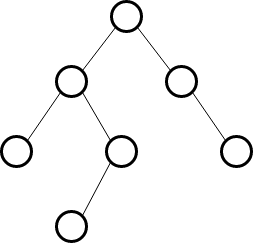Que – 2. What is the worst case possible height of AVL tree?
(A) 2*logn
(B) 1.44*log n
(C) Depends upon implementation
(D) θ(n)

Solution: The worst case possible height of AVL tree with n nodes is 1.44*logn. This can be verified using AVL tree having 7 nodes and maximum height.Checking for option (A), 2*log7 = 5.6, however height of tree is 3.
Checking for option (B), 1.44*log7 = 4, which is near to 3.
Checking for option (D), n = 7, however height of tree is 3.
Out of these, option (B) is the best possible answer.

Type 2: Based on complexity of insertion, deletion and searching in AVL tree –

Que – 3. Which of the following is TRUE?
(A) The cost of searching an AVL tree is θ(log n) but that of a binary search tree is O(n)
(B) The cost of searching an AVL tree is θ(log n) but that of a complete binary tree is θ(n log n)
(C) The cost of searching a binary search tree is O(log n ) but that of an AVL tree is θ(n)
(D) The cost of searching an AVL tree is θ(n log n) but that of a binary search tree is O(n)

Solution: AVL tree’s time complexity of searching, insertion and deletion = O(logn). But a binary search tree, may be skewed tree, so in worst case BST searching, insertion and deletion complexity = O(n).

Que – 4. The worst case running time to search for an element in a balanced in a binary search tree with n*2^n elements is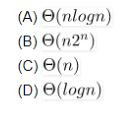Solution: Time taken to search an element is Θ(logn) where n is number of elements in AVL tree.
As number of elements given is n*2^n, the searching complexity will be Θ(log(n*2^n)) which can be written as:

```= Θ(log(n*2^n))
= Θ(log(n)) + Θ(log(2^n))
= Θ(log(n)) + Θ(nlog(2))
= Θ(log(n)) + Θ(n)
```

As logn is asymptotically smaller than n, Θ(log(n)) + Θ(n) can be written as Θ(n) which matches option C.

Type 3: Insertion and Deletion in AVL tree –
The question can be asked on the resultant tree when keys are inserted or deleted from AVL tree. Appropriate rotations need to be made if balance factor is disturbed.

Que – 5. Consider the following AVL tree.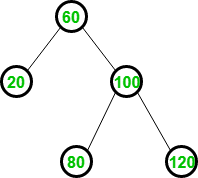Which of the following is updated AVL tree after insertion of 70?

(A)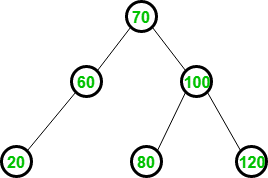(B)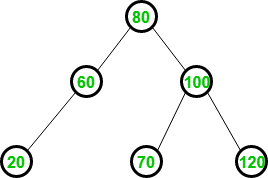(C)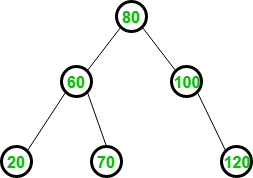(D) None

Solution: The element is first inserted in the same way as BST. Therefore after insertion of 70, BST can be shown as: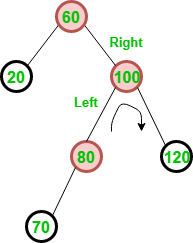However, balance factor is disturbed requiring RL rotation. To remove RL rotation, it is first converted into RR rotation as: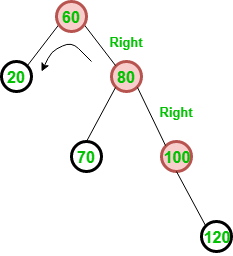After removal of RR rotation, AVL tree generated is same as option (C).My Personal Notes arrow_drop_up

This article is contributed by Sonal Tuteja. If you like GeeksforGeeks and would like to contribute, you can also write an article using contribute.geeksforgeeks.org or mail your article to contribute@geeksforgeeks.org. See your article appearing on the GeeksforGeeks main page and help other Geeks.

Please Improve this article if you find anything incorrect by clicking on the "Improve Article" button below.

Article Tags :
Practice Tags :

12

Please write to us at contribute@geeksforgeeks.org to report any issue with the above content.